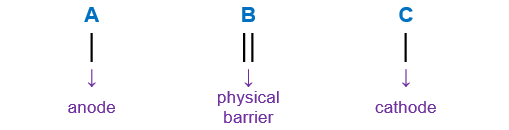# Problem: Write the overall balanced equation for the reaction.Sn(s) | Sn2+(aq) || NO(g) | NO3-(aq),H+(aq) | Pt(s)Write the overall balanced equation for the reaction.a. 3Sn(s) + NO3-(aq) + 8H+(aq) ---&gt; 3Sn2+(aq) + 2NO(g) + H2O(l)b. Sn(s) + 2NO3-(aq) + 4H+(aq) ---&gt; Sn2+(aq) + NO(g) + 2H2O(l)c. 3Sn(s) + 2NO3-(aq) + 8H+(aq) ---&gt; 3Sn2+(aq) + 2NO(g) + 4H2O(l)d. Sn(s) + NO3-(aq) + 4H+(aq) ---&gt; Sn2+(aq) + NO(g) + 2H2O(l)

###### FREE Expert Solution

We’re being asked to write the overall balanced equation for the given cell notation below:

Sn(s) | Sn2+(aq) || NO(g) | NO3-(aq), H+(aq) | Pt(s)

When writing a cell notation, we use the following format – “as easy as ABCRecall the mnemonics LEO GER.###### Problem Details

Write the overall balanced equation for the reaction.

Sn(s) | Sn2+(aq) || NO(g) | NO3-(aq),H+(aq) | Pt(s)

Write the overall balanced equation for the reaction.

a. 3Sn(s) + NO3-(aq) + 8H+(aq) ---> 3Sn2+(aq) + 2NO(g) + H2O(l)

b. Sn(s) + 2NO3-(aq) + 4H+(aq) ---> Sn2+(aq) + NO(g) + 2H2O(l)

c. 3Sn(s) + 2NO3-(aq) + 8H+(aq) ---> 3Sn2+(aq) + 2NO(g) + 4H2O(l)

d. Sn(s) + NO3-(aq) + 4H+(aq) ---> Sn2+(aq) + NO(g) + 2H2O(l)

What scientific concept do you need to know in order to solve this problem?

Our tutors have indicated that to solve this problem you will need to apply the Cell Notation concept. If you need more Cell Notation practice, you can also practice Cell Notation practice problems.

What is the difficulty of this problem?

Our tutors rated the difficulty ofWrite the overall balanced equation for the reaction.Sn(s) |...as medium difficulty.

How long does this problem take to solve?

Our expert Chemistry tutor, Sabrina took 8 minutes and 23 seconds to solve this problem. You can follow their steps in the video explanation above.

What professor is this problem relevant for?

Based on our data, we think this problem is relevant for Professor Jones' class at University of Guelph.